/

### Function Description

Returns an array providing the result of applying an exponential smoothing algorithm to the InputArray (algorithm used is compatible with the one implicit in the Excel Data Analysis Toolpack as at 2012).

If the input array,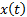, is of lengththen the output consists of a two dimensional array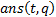of size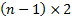where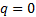corresponds to the exponentially smoothed values,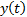and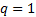corresponds to the “standard error” of these values,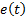, these being calculated as follows, where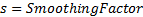and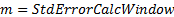: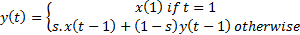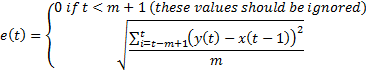Contents | Prev | Next